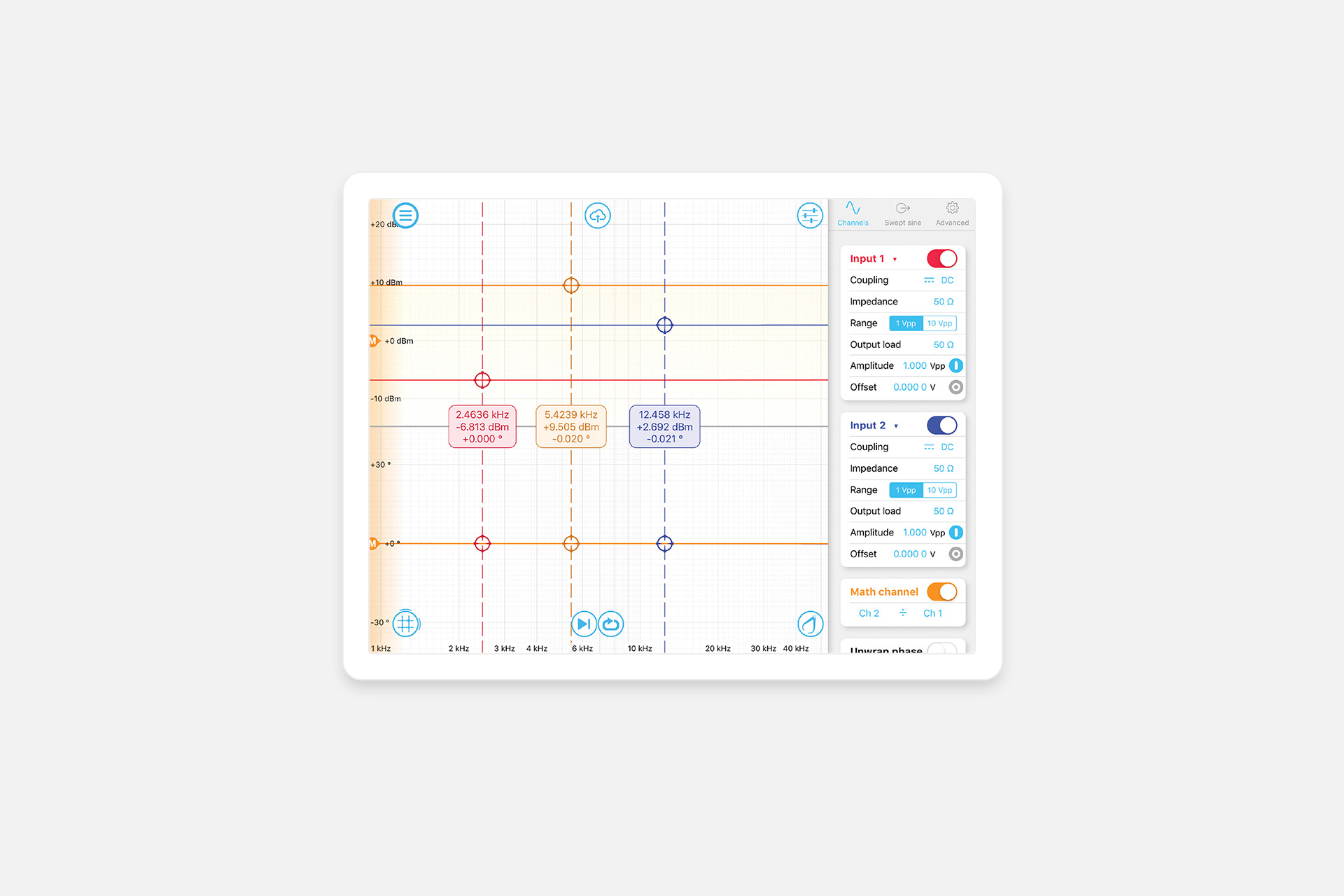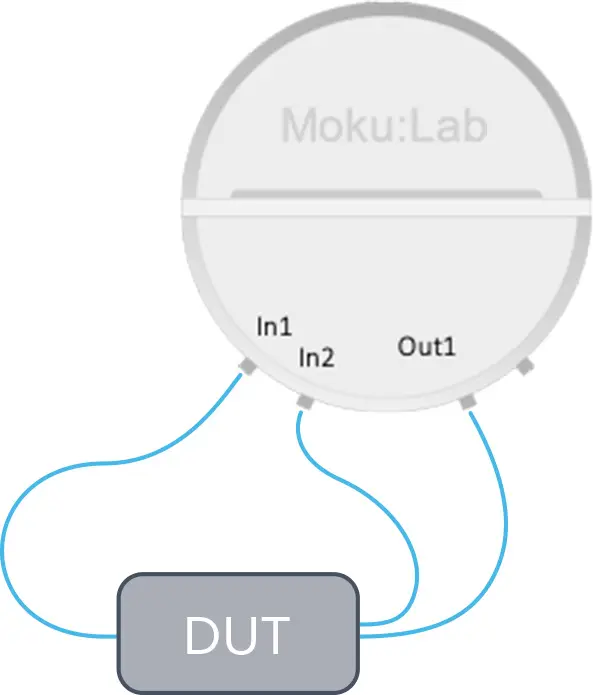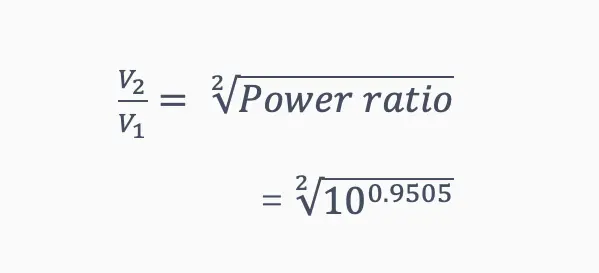Application Notes

# Impedance Measurements — A guide to measuring impedance with Moku:Lab’s Frequency Response Analyzer (Part 1)In this application note, we present some examples of making accurate impedance measurements with Moku:Lab. In this first part, we will explore the maths of measuring resistance and accuracy. In the second part, we expand to explore capacitive and inductive measurements.

## Frequency Response Analyzer

Moku:Lab’s Frequency Response Analyzer (FRA) drives a swept sine wave on the Moku outputs and simultaneously measures the received signal amplitude (or power) on the Moku input. This can be used to measure the transfer function of a system or (device under test) and thus create a plot of amplitude and phase vs frequency, commonly referred to as a Bode plot.

## Power units

In order to measure the impedance of a device under test (Zdut), we need to understand the power plots of the FRA. The FRA plots use units of dBm, or decibels relative to one milliwatt (1mW); a convenient unit of measure in this situation. Defined as:Moku:Lab’s FRA swept sine output can be set in volts (peak to peak) or dBm. We will use volts for the output. By definition for a sinusoid,substituting for Vrms in (2) givesSo 1 Vpp expressed in dBm, scaling for mW and knowing the Moku:Lab input impedance is 50Ω gives:Figure 1 shows use of the FRA to generate a 1 Vpp sine wave and with Moku:Lab’s output 1 connected via direct coax to input 1. The resulting amplitude is of course flat across the frequency range (0-1kHz) and at 4.050dBm, very close to the calculated 3.979dBm. The discrepancy equates to 1.7mV (or 0.17%).Figure 1: FRA plot of 1 Vpp driven directly in Moku:Lab’s input

## Impedance

#### Single port measurement:

Now that the FRA power units are understood we can measure an impedance.

In this first example, we are going to measure a Rdut of a simple 10k ohm, 10% tolerance resistor. The effective circuit is:Figure 2: Equivalent circuit

Note, Vout is 2v, since this results in 1V across load of 50Ω.

Moku:Lab’s FRA operates up to 120MHz, but for these resistance measurements, a plot to 40kHz is sufficient. Figure 3 shows Moku:Lab’s FRA amplitude response = -35.821 dBm at Vin.Figure 3: FRA of 10kΩ DUT, single port

Rearranging power equation (1) and substituting P from (4) we can state:With measured Pdb of -35.821dBm, we calculate Vin= 10.23mV.

The resistor divider of Rdut and Moku:Lab’s 50Ω inputs and output in figure 2 gives us:ThusSolving gives Rdut = 9675 ohms.

A DVM reading of this resistor showed 9750 ohms.

So from this simple, one resistor measurement we can conclude Moku:Lab is accurate within 77 ohms (< 1%).

#### Low impedance measurement:

The example above used a standard 10% tolerance resistor. We are now interested in measuring a lower impedance to a high level of accuracy. To do this we will use a 100 ohm, 0.005% tolerance high precision resistor. Using the above method we obtain a power magnitude plot (Figure 4)Figure 4: FRA of 100Ω, 0.005%, single port

Applying the measured power of -1.972 dBm to equations (5) & (7) we calculate Rdut to be 98.41 ohms. This agrees with the known value, but we can do better with a two port measurement.

#### Two-port measurement:

In order to have an improved measurement, we need to account for the loading of the DUT on the Moku’s 50ohm output.

We can accomplish this with a two-port measurement whereby we utilize the second input port of the Moku to observe the actual applied signal level.Figure 5: Two-port configurationFigure 6: Two-port equivalent circuit

We can derive Rdut in figure 6, from ohms law:Substitute (9) into (8)orWe set up this two-port measurement with our tight tolerance 100Ω, 0.005% resistor and captured the Moku:Lab’s FRA plot in Figure 7.Figure 7: 100 ohm, two-port

Note, we have used the Frequency Response Analyzer math channel to produce V2/V1. This is very quick and simple to configure on the iPad interface.

From (10) we see that the Rdut can be calculated simply from the V2:V1 voltage ratio.

The Frequency Response Analyzer math channel has calculated the power ratio as 9.505 dBm and thus the voltage ratio is:So we can apply (10) to obtain Rdut =99.36 ohms.

We can now apply this 2 port method to the original 10k / 20% resistor; Figure 8 shows the FRA responseFigure 8: 10k ohm, two-port

Using our established formula; power ratio of 45.856 dBm gives Rdut = 9762 ohms. A useful improvement.

## Summary

The Moku:Lab FRA can be used to make impedance measurements and determine to a <1% accuracy, the value of resistance.The two-port method allows for the loading of the DUT and appears more accurate.

Now that this method and calculations are explained, in part 2 we extend the examples to using the FRA to measure complex impedance, capacitance & inductance, and to more complex systems and across frequency.

Thanks for reading. You can download the Moku:Lab App from Apple’s App Store and experience it in demo mode. Specific features of Moku:Lab’s FRA can be found in the FRA User Manual.